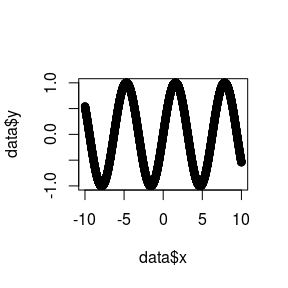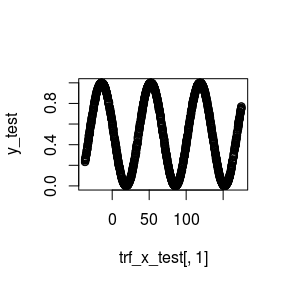# nntrf for regression

#### 2021-02-26

library(nntrf)
library(mlr)
#> 'mlr' is in maintenance mode since July 2019. Future development
#> efforts will go into its successor 'mlr3'
#> (<https://mlr3.mlr-org.com>).
library(mlrCPO)
library(FNN)

# Overview

Here, nntrf is going to be applied to a simple regression problem $$y = sin(x)$$, with a single relevant attribute (x). 8 random attributes will be added, and then the instances will be randomly rotated, so that none of the 10 attributes contains all the information. Then, nntrf will be used to transform this feature space and knn will be used as machine learning method on this space.

Let’s first create the regression data.

x <- seq(-10,10,length.out = 5000)
y <- sin(x)
extra <- matrix(runif(5000*9),nrow = 5000)
data <- cbind(x, as.data.frame(extra), y)
plot(data$x,data$y)Now, let’s add 9 random attributes to the dataset, and rotate every instance with a random rotation matrix. The result is that none of the 10 attributes can now be identified with the only relevant attribute (originally, x).

m <- as.matrix(cbind(x, extra))
set.seed(0)
mat_ortho <- pracma::randortho(ncol(m), type = c("orthonormal"))
m_p <- m %*% mat_ortho
data <- cbind(as.data.frame(m_p), y)

Inputs and output are normalized to the 0-1 range using mlr utilities.

data <- mlr::normalizeFeatures(data, method="range")

Data is now divided into train / test partitions.

rd <- data
n <- nrow(rd)

set.seed(0)
training_index <- sample(1:n, round(0.6*n))

train <- rd[training_index,]
test <- rd[-training_index,]
x_train <- train[,-ncol(train)]
y_train <- train[,ncol(train)]
x_test <- test[,-ncol(test)]
y_test <- test[,ncol(test)] 

Now, let’s see the MAE error of the original (x,y) instances (prior to adding the random attributes and performing the random rotation). This is the best result we could expect from KNN (having fixed the number of neighbors to k=3).

set.seed(0)
outputs <- FNN::knn.reg(as.data.frame(x[training_index]), as.data.frame(x[-training_index]), y_train, k=3)
mae_error <- mean(abs(outputs$pred - y_test)) cat(paste0("MAE of KNN (K=3):", mae_error, "\n")) #> MAE of KNN (K=3):0.00107947968440619 Next, test MAE is computed with the randomized dataset (+9 random attributes and random rotation). It is much worse than the one above. set.seed(0) outputs <- FNN::knn.reg(x_train, x_test, y_train, k=3) mae_error <- mean(abs(outputs$pred - y_test))
cat(paste0("MAE of KNN (K=3):", mae_error, "\n"))
#> MAE of KNN (K=3):0.117339310780657

Next, nntrf is used to reduce the 10 dimensions to the 1 relevant dimension. It can be seen that MAE improves significantly over previous result, if no sigmoid is used in the nntrf transformation (however, it is still somewhat far away from the optimal result).

set.seed(0)
nnpo <- nntrf(formula=y~.,
data=train,
size=1, maxit=500, trace=FALSE, repetitions=2)

# With sigmoid

trf_x_train <- nnpo$trf(x=x_train,use_sigmoid=TRUE) trf_x_test <- nnpo$trf(x=x_test,use_sigmoid=TRUE)

outputs <- FNN::knn.reg(trf_x_train, trf_x_test, y_train, k = 3)
mae <- mean(abs(outputs$pred - y_test)) cat(paste0("MAE of KNN (K=3) transformed by nntrf with Sigmoid: ", mae, "\n")) #> MAE of KNN (K=3) transformed by nntrf with Sigmoid: 0.215157453152229 # With no sigmoid trf_x_train <- nnpo$trf(x=x_train,use_sigmoid=FALSE)
trf_x_test <- nnpo$trf(x=x_test,use_sigmoid=FALSE) outputs <- FNN::knn.reg(trf_x_train, trf_x_test, y_train, k=3) mae <- mean(abs(outputs$pred - y_test))
cat(paste0("MAE of KNN (K=3) transformed by nntrf with no sigmoid: ", mae, "\n"))
#> MAE of KNN (K=3) transformed by nntrf with no sigmoid: 0.00690412204721888

It can be seen that the sine shape was restored.

plot(trf_x_test[,1], y_test)Now, let’s use hyper-parameter tuning, with 3-fold crossvalidation for model evaluation and 3-fold crossvalidation for hyper-parameter tuning.

sin_task <- makeRegrTask(data=data, target="y")

control_grid <- makeTuneControlGrid()
inner_desc <- makeResampleDesc("CV", iter=3)
outer_desc <-  makeResampleDesc("CV", iter=3)
set.seed(0)
outer_inst <- makeResampleInstance(outer_desc, sin_task)

What follows is the definition of nntrf as a pre-processing stage for mlr. It can be just copied.

cpo_nntrf = makeCPO("nntrfCPO",
# Here, the hyper-parameters of nntrf are defined
pSS(repetitions = 1 : integer[1, ],
size: integer[1, ],
maxit = 100 : integer[1, ],
use_sigmoid = FALSE: logical),
dataformat = "numeric",
cpo.train = function(data, target,
repetitions,
size, maxit, use_sigmoid) {
data_and_class <- cbind(as.data.frame(data), class=target[])
nnpo <- nntrf(repetitions=repetitions,
formula=class~.,
data=data_and_class,
size=size, maxit=maxit, trace=FALSE)
},
cpo.retrafo = function(data, control,
repetitions,
size, maxit, use_sigmoid) {

trf_x <- control$trf(x=data,use_sigmoid=use_sigmoid) trf_x }) Next, the pipeline of pre-processing + classifier method (KNN in this case) is defined. # knn is the machine learning method. The knn available in the FNN package is used knn_lrn <- makeLearner("regr.fnn") # Then, knn is combined with nntrf's preprocessing into a pipeline knn_nntrf <- cpo_nntrf() %>>% knn_lrn # Just in case, we fix the values of the hyper-parameters that we do not require to optimize # (not necessary, because they already have default values. Just to make their values explicit) knn_nntrf <- setHyperPars(knn_nntrf, nntrfCPO.repetitions=1, nntrfCPO.maxit=100, nntrfCPO.use_sigmoid=FALSE) # However, we are going to use 2 repetitions here, instead of 1 (the default): knn_nntrf <- setHyperPars(knn_nntrf, nntrfCPO.repetitions=2) Next, the hyper-parameter space for the pipeline is defined. Only three hyper-parameters will be optimized: the number of KNN neighbors (k), from 1 to 7, the number of hidden neurons (size), from 1 to 5, and the number of iterations. The remaining hyper-parameters are left to some default values. ps <- makeParamSet(makeDiscreteParam("k", values = 1:7), makeDiscreteParam("nntrfCPO.size", values = 1:5), makeDiscreteParam("nntrfCPO.maxit", values = c(50, 100, 500, 1000, 2000)) ) Next, a mlr wrapper is used to give the knn_nntrf pipeline the ability to do hyper-parameter tuning. knn_nntrf_tune <- makeTuneWrapper(knn_nntrf, resampling = inner_desc, par.set = ps, control = control_grid, measures = list(mlr::mae), show.info = FALSE) Finally, the complete process (3-fold hyper-parameter tuning) and 3-fold outer model evaluation is run. It takes some time. set.seed(0) # Please, note that in order to save time, results have been precomputed cached <- system.file("extdata", "error_knn_nntrf_regression.rda", package = "nntrf") if(file.exists(cached)){load(cached)} else { error_knn_nntrf_tune <- resample(knn_nntrf_tune, sin_task, outer_inst, measures = list(mlr::mae), extract = getTuneResult, show.info = FALSE) # save(error_knn_nntrf_tune, file="../inst/extdata/error_knn_nntrf_regression.rda") } Errors (mae.test.mean) and optimal hyper-parameters are printed below. It can be seen that hyper-parameter tuning is not able to detect that only 1 relevant attribute is needed, but MAE test results will show (later) that the projection carried out by nntrf achieves a good MAE result. print(error_knn_nntrf_tune$extract)
#> []
#> Tune result:
#> Op. pars: k=4; nntrfCPO.size=5; nntrfCPO.maxit=2000
#> mae.test.mean=0.0023038
#>
#> []
#> Tune result:
#> Op. pars: k=6; nntrfCPO.size=5; nntrfCPO.maxit=500
#> mae.test.mean=0.0023746
#>
#> []
#> Tune result:
#> Op. pars: k=2; nntrfCPO.size=5; nntrfCPO.maxit=500
#> mae.test.mean=0.0023567

The final outer 3-fold crossvalition accuracy is displayed below. Please, note that this mae.test.mean corresponds to the outer 3-fold crossvalidation, while the mae.test.mean above, corresponds to the inner 3-fold crossvalidation mae (computed during hyper-parameter tuning). It can be seen that, while hyper-parameter tuning was not able to reduce the feature space to just 1 attribute (it was reduced to 5 attributes), the test MAE obtained is reasonable.

print(error_knn_nntrf_tune$aggr) #> mae.test.mean #> 0.001877029 ## Hyper-parameter tuning with PCA In order to compare a supervised transformation method (nntrf) with an unsupervised one (PCA), it is very easy to do exactly the same pre-processing with PCA. In this case, the main hyper-parameters are k (number of KNN neighbors) and Pca.rank (the number of PCA components to be used, which would be the counterpart of size, the number of hidden neurons used by nntrf). knn_pca <- cpoPca(center=TRUE, scale=TRUE, export=c("rank")) %>>% makeLearner("regr.fnn") ps_pca <- makeParamSet(makeDiscreteParam("k", values = 1:7), makeDiscreteParam("pca.rank", values = 1:5) ) knn_pca_tune <- makeTuneWrapper(knn_pca, resampling = inner_desc, par.set = ps_pca, control = control_grid, measures = list(mlr::mae), show.info = FALSE) set.seed(0) # Please, note that in order to save time, results have been precomputed cached <- system.file("extdata", "error_knn_pca_tune_regression.rda", package = "nntrf") if(file.exists(cached)){load(cached)} else { error_knn_pca_tune <- resample(knn_pca_tune, sin_task, outer_inst, measures = list(mlr::mae), extract = getTuneResult, show.info = FALSE) #save(error_knn_pca_tune, file="../inst/extdata/error_knn_pca_tune_regression.rda") } The cell below displays the results obtained by hyper-parameter tuning for the three external crossvalidation folds. print(error_knn_pca_tune$extract)
#> []
#> Tune result:
#> Op. pars: k=6; pca.rank=5
#> mae.test.mean=0.1064710
#>
#> []
#> Tune result:
#> Op. pars: k=5; pca.rank=5
#> mae.test.mean=0.1057195
#>
#> []
#> Tune result:
#> Op. pars: k=4; pca.rank=5
#> mae.test.mean=0.1068931

It can be seen below that PCA obtains a much worse MAE than nntrf, if forced to use no more than 5 components.

print(error_knn_pca_tune$aggr) #> mae.test.mean #> 0.1006023 ## Hyper-parameter tuning with just KNN For completeness sake, below are the results with no pre-processing, just KNN (results are better than those of PCA with only 5 components) but worse than nntrf). ps_knn <- makeParamSet(makeDiscreteParam("k", values = 1:7)) knn_lrn <- makeLearner("regr.fnn") knn_tune <- makeTuneWrapper(knn_lrn, resampling = inner_desc, par.set = ps_knn, control = control_grid, measures = list(mlr::mae), show.info = FALSE) set.seed(0) # Please, note that in order to save time, results have been precomputed cached <- system.file("extdata", "error_knn_tune_regression.rda", package = "nntrf") if(file.exists(cached)){load(cached)} else { error_knn_tune <- resample(knn_tune, sin_task, outer_inst, measures = list(mlr::mae), extract = getTuneResult, show.info = FALSE) #save(error_knn_tune, file="../inst/extdata/error_knn_tune_regression.rda") } print(error_knn_tune$aggr)
#> mae.test.mean
#>    0.07660643